# Array sorting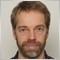42493

I am developing an Expert Advisor where I would like to implement FIFO support (closing orders according to order open time, sorted ASC). It it possible to use a similar logic described in this article: https://www.mql5.com/en/forum/138127.

It does not seems very straightforwarded though. My question is if it would be possible to use the ArraySort() function instead (https://docs.mql4.com/array/arraysort). It can only sort numerical arrays but if I understand it correctly datetime values will be stored as integers (number of seconds since 01.01.1970). Hence a numerical value.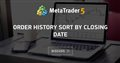Order History sort by closing date
• 2012.02.21
• www.mql5.com
Hello, i want to build a simple indicator about my profit-loss analysis... as Select by pos is related to Ticket ID/Order Date...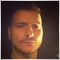18914

Yes , they will become integers , you just create a temporary 2 dimensional integer array with 2 end nodes []

Firts pass you enter the time on node [x] and the ticket (or index in your managing array) on node [x]
You sort this array (it will be sorted based on [x] values ) and you essentially get sorted list with pointers to tickets or indices on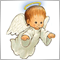3025

Jan Flodin:

I am developing an Expert Advisor where I would like to implement FIFO support (closing orders according to order open time, sorted ASC). It it possible to use a similar logic described in this article: https://www.mql5.com/en/forum/138127.

It does not seems very straightforwarded though. My question is if it would be possible to use the ArraySort() function instead (https://docs.mql4.com/array/arraysort). It can only sort numerical arrays but if I understand it correctly datetime values will be stored as integers (number of seconds since 01.01.1970). Hence a numerical value.

You can use the ArraySort() function for this purpose. But are you sure you want to sort whole array if you only need to implement FIFO? Isn't enough to find the oldest trade? Of course it depends on your purpose.3025

Lorentzos Roussos:
Yes , they will become integers , you just create a temporary 2 dimensional integer array with 2 end nodes []

If you mean long as integer type then it's alright.42493

Lorentzos Roussos:
Yes , they will become integers , you just create a temporary 2 dimensional integer array with 2 end nodes []

Firts pass you enter the time on node [x] and the ticket (or index in your managing array) on node [x]
You sort this array (it will be sorted based on [x] values ) and you essentially get sorted list with pointers to tickets or indices on

Thanks for the tip. I think that only values in the first dimension can be sorted, i.e. the values you put in [x]. But I might be mistaken.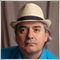4983

Jan Flodin: Thanks for the tip. I think that only values in the first dimension can be sorted, i.e. the values you put in [x]. But I might be mistaken.

There is no need to "sort". A sort is already a repetitive, iterative procedure that goes over the data several times in order to sort the data, one element at a time.

So you might as well do a similar thing directly, which is to find the oldest order and close it, and then repeat if necessary. As you scan, you just have to keep track of a date-time variable and a ticket number.

Here is some pseudo code:

```Do

FIFOTicket    = WRONG_VALUE
FIFOTimestamp = WRONG_VALUE

For i = (TradeCount - 1) to 0

If ( ( Trade[i].Timestamp < FIFOTimestamp ) or ( FIFOTicket == WRONG_VALUE ) ) Then

Next i

If ( FIFOTicket != WRONG_VALUE ) Then CloseTrade( FIFOTicket )

While ( FIFOTicket != WRONG_VALUE )```3025

Jan Flodin:

Thanks for the tip. I think that only values in the first dimension can be sorted, i.e. the values you put in [x]. But I might be mistaken.

Lorentzos are right. You can try this example code:

```void OnStart()
{
long arr={{0,0},{0,0},{0,0},{0,0},{0,0}};
for(int i=0;i<5;i++)
{
arr[i,1]=i+1;
}
for(int i=0;i<5;i++)
printf("Unsorted ticket %I64d = %s",arr[i,1],TimeToString(arr[i,0],TIME_DATE|TIME_MINUTES|TIME_SECONDS));
ArraySort(arr);
for(int i=0;i<5;i++)
printf("Sorted ticket by date %I64d = %s",arr[i,1],TimeToString(arr[i,0],TIME_DATE|TIME_MINUTES|TIME_SECONDS));
}```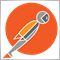2392

IMHO, sorting two-dimensional static arrays feels a dirty hack that takes me back to the pre-600 days. I think a better question which would lead to a more sustainable pattern would be, "how do you sort a collection of objects in MQL?"

Almost all classes in the standard library inherit the universal base class, CObject. CObject provides a virtual comparative method which is called from the collections for quick-sorting. So all you have to do is subclass CObject (or descendant) and override the Compare method. Then you can easily sort any object from any collection type. In this example I'm using a simple custom collection I call objvector.

```#include <Arrays\ArrayObj.mqh>
template <typename T>
class objvector : public CArrayObj
{
public:
T operator[](const int index) const { return this.At(index); }
bool InsertSort(T element) { this.Sort(); return CArrayObj::InsertSort(element); }
};

{
protected:
int      m_ticket;
datetime m_open_time;
public:
if(this.select())
m_open_time = ::OrderOpenTime();
else
m_open_time = INT_MAX;
}
bool select() const {
return ::OrderSelect(m_ticket, SELECT_BY_TICKET);
}
virtual int Compare(const CObject *node, const int mode=0) const override {
if(this.m_open_time > other.m_open_time)
return 1;
if(this.m_open_time < other.m_open_time)
return -1;
return 0;
}
}; ```

This may seem like a lot more work, but once you add this to your lib you can then quickly sublass it and add more attributes, methods, and sorting modes without having to refactor your entire algo. It will save you so much time in the bigger-picture, and your code will be much more readable.

```void OnStart()
{
for(int i=OrdersHistoryTotal()-1; i>=0; --i)
if(OrderSelect(i, SELECT_BY_POS, MODE_HISTORY) && OrderType() < 2)
Print(__FUNCTION__," Error: ", _LastError);

for(int i=0; i<total; i++)
printf("%d opened at %s",
OrderTicket(),
TimeToString(OrderOpenTime())
);
}```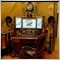494

Jan Flodin:

I am developing an Expert Advisor where I would like to implement FIFO support (closing orders according to order open time, sorted ASC). It it possible to use a similar logic described in this article: https://www.mql5.com/en/forum/138127.

It does not seems very straightforwarded though. My question is if it would be possible to use the ArraySort() function instead (https://docs.mql4.com/array/arraysort). It can only sort numerical arrays but if I understand it correctly datetime values will be stored as integers (number of seconds since 01.01.1970). Hence a numerical value.

```//+------------------------------------------------------------------+
//|                                                                  |
//+------------------------------------------------------------------+
void Close_AllOrders()
{
/*-----------------------------------------------------------------------------------------------*/
//Close Orders according to FIFO Rule
for(i=0; i<OrdersTotal(); i++)
{
if(OrderSymbol()==Symbol())
{
{
Close_Result=OrderClose(OrderTicket(),OrderLots(),Bid,0,clrNONE);
if(Close_Result) i--;
}
if(OrderType()==OP_SELL)
{
if(Close_Result) i--;
}
}
}
/*-----------------------------------------------------------------------------------------------*/
//Error Control
GetLastError();
if(GetLastError()>=3)
{
Print("Close_AllOrders() ..Error  : "+ErrorDescription(GetLastError()));
}
/*-----------------------------------------------------------------------------------------------*/
}```

Why sort when you can close them with a simple function?2392

Donald Gibson:

Why sort when you can close them with a simple function?

FIFO orders have to be closed in the order they were opened. Your function would (kind-of) work on a normal hedging account, but would likely fail under FIFO conditions. Also, you never want to iterate the order pool forward when closing orders because the order pool will shift as you close orders and you will skip orders. Instead, you always need to iterate in reverse.

Addressing your sorting question, sorting is necessary for any other calculations that involve a sorted order pool that doesn't involve exclusively closing orders. And finally, a Fifo-CloseAll function could be further simplified thru recursion.

```bool fifo_positions_closeall(const string symbol=NULL, const int magic=INT_MAX)
{
int ticket = -1;
datetime open_time = -1;
for(int i=OrdersTotal()-1; i>=0; --i){
if(   OrderSelect(i, SELECT_BY_POS)
&& OrderType() < 2
&& (symbol == NULL || symbol == OrderSymbol())
&& (magic == INT_MAX || magic == OrderMagicNumber())
&& (open_time == -1 || OrderOpenTime() < open_time)
){
ticket = OrderTicket();
open_time = OrderOpenTime();
}
}
if(OrderSelect(ticket, SELECT_BY_TICKET)) {
RefreshRates();
if(OrderClose(ticket, OrderLots(), OrderClosePrice(), 10)) {
return fifo_positions_closeall(symbol, magic);
}else{
return false;
}
}
return true;
}```42493

Thanks a lot all of you for your help:-)

Each solution has its advantage. Depends on the situation. For my case I will use the custom collection suggested by Nicholi.

Jan